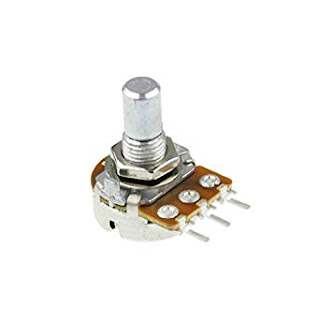# Basic Electronic Components | All About Resistor

### Basic Electronic Components | All About Resistor

The resistor is very useful component in electronic circuits. You will see resistors everywhere in electronic circuits. Why we use resistors in every electronic circuit? We use the resistor to control the voltages and the currents in our circuits.

Let’s say that, you have a 12V battery and you want to turn on an 5v LED. If you connect the
battery directly to the LED, 12v of the battery will fl ow through the LED! but LED required voltages are 5v so, after some time the LED will become very hot or burn out.

But, if you put a resistor in series with the LED, you can get required voltages for Your LED

Resistor Symbole Used in Diagrams

A common way to use resistor is to control the current for the LED

A standard LED can handle only up to 20 to 30 mA around. If the current it much bigger than that,
the LED will be die quickly.

Let’s say that, the LED in circuit above needs 15 mA to give a better light, and it has a
voltages drop of 2 volts. (These values should be specified by the supplier when

If anyone have a 9 Volt battery that, you would like to power it with which resistor value
do you need?

The voltage specified for LED is the voltage drop the LED will have under normal condition.
That mean you know you will have 2V over the LED.

Read Also: 250+ LCD LED Penal COF IC Datasheets

Types Of Resistor

There of much types of resistor but I will show main types of resistor, So there are below some types of resistor.

Fixed Value Resistor

This is fixed value resistor. You can not change it's value because it is fixed value resistor.Fixed ResistorFixed Resistor Circuit Symbol

Variable Resistor

This type of resistor is variable resistor that means, you can change it's value easily. You can set your required value.Variable ResistorVariable Resistor Circuit Symbole

Trimmer Resistor

This is used in Electronic equipments to make small adjustments in circuitsTrimmer ResistorTrimmer Resistor Circuit Symbol

Rheostat Resistor

This is a High wattage variable resistor. It is used to limit the current flow their power rating is higherRheostat Resistor

Varistor Resistor

The non ohmic variable resistor is known as varistor. This is the special type of resistorVaristor ResistorVaristor Resistor Symbol

This Post Will Update Time to Time